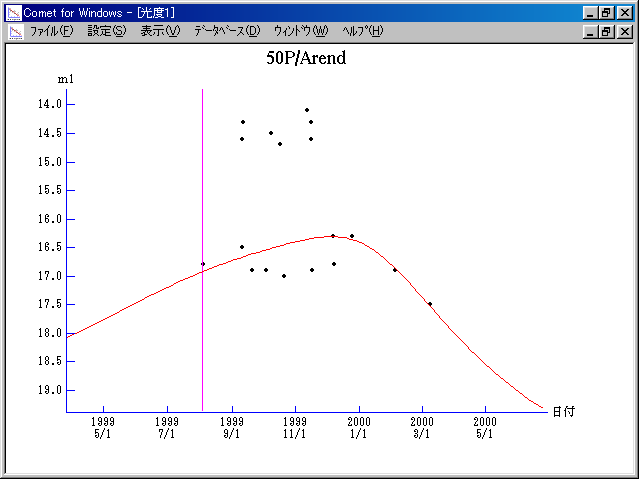# \$B%"%i%sWB@1(B

50P/Arend (1999)###\$B###\$B50F;MWAG(B

```   The following improved orbital elements by Kenji Muraoka,
are from 114 observations 1951 to 2000, including 9 Planets,
Moon and 5 minor planets perturbations and non-gravitational
effect of style II.  The mean residual is +/- 1.11 arc seconds.

Epoch  =  1999 Aug. 10.0  TT       JDT = 2451400.5
T  =  1999 Aug.  3.76842       +/- 0.00072 (m.e.) TT
Peri. =   48.99390                +/- 0.00038
Node  =  355.38259                +/- 0.00011   (2000.0)
Incl. =   19.17917                +/- 0.00003
q  =    1.9166945              +/- 0.0000020 AU
e  =    0.5303522              +/- 0.0000005
a  =    4.0811319              +/- 0.0000015 AU
n  =    0.11954547             +/- 0.00000007
P  =    8.245                  +/- 0.0000046  years
A1  =   +0.059                  +/- 0.029
A2  =   -0.02235                +/- 0.00043
```

###\$B@1?^(B###\$B8wEYJQ2=(B

```        m1 = 13.8 + 5 log\$B&\$(B + 5.0 log r
```##### \$B50F;MWAG\$OB<2,7r<#;a\$N7W;;\$K\$h\$k\$b\$N\$G\$9!#(B \$B@1?^\$O(B StellaNavigator Ver.2.0 for Windows (\$B%"%9%H%m%"!<%D(B \$BJTCx(B / \$B%"%9%-!<=PHG6I4)(B) \$B\$G:n@.\$7\$?\$b\$N\$G\$9!#(B \$B8wEY%0%i%U\$O(BComet for Windows\$B\$G:n@.\$7\$?\$b\$N\$G\$9!#(B